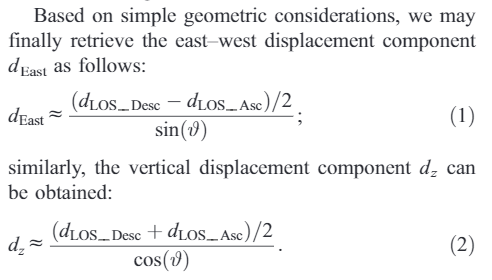# E-W and vertical displacements

Hello everyone,
I’m working on DInSAR for the detection of ground deformation induced by large earthquakes, and I’m facing the issue of the retrieval of E-W displacement and vertical displacement. I read a lot of topics here in the forum and also I’ve studied a lot of papers, and my approach follows what is proposed in Manzo et al. (2006):So, first of all I performed these steps:

• TOPSAR split
• apply orbit file
• Back geocoding
• Enhanced spectral diversity
• interferogram formation with topographic phase removal
• deburst
• goldstein filter
• snaphu unwrapping
• cut of low coherence pixels on the unwrapped phase (coh > 0.3)
• phase to displacement
• find a portion assumed with no-displacement and subtraction of the value from the phase displacement product
This workflow has been reproduced in two couples of data with small baseline, one for ascending and one for descending orbits, and then in BandMaths I reproduce the approach by writing:

FOR E-W COMPONENT: ((((\$5.displacement - \$6.displacement) / 2) / (sin(rad(\$6.incident_angle)))))
FOR VERTICAL COMPONENT: ((((\$5.displacement + \$6.displacement) / 2) / (cos(rad(\$6.incident_angle)))))

with \$5.displacement = descending orbit displacement
and \$6.displacement = ascending orbit displacement
In this example I used the incident angle from descending orbit, but using the one contained in the tie point grid of ascending orbit data, the result is the same. Then, at the end, I perform Terrain Correction.

What I obtain is not what I expect, comparing my results with those in bibliography. Or better, the E-W component is approximatively comparable, while the vertical is completely different. For the vertical component, I followed other approaches suggested on the forum, as for example the Pythagora theorem for the triangles: in this way, I multiply the cos(rad(incident_angle)) with the LOS displacement for both orbits and average them ((vert_desc + vert_asc)/2) but the product is still not good. Moreover, the result is with opposite sign in respect with the case study with which I’m comparing my results.

where am I wrong? Does anyone can help me on following other approaches?